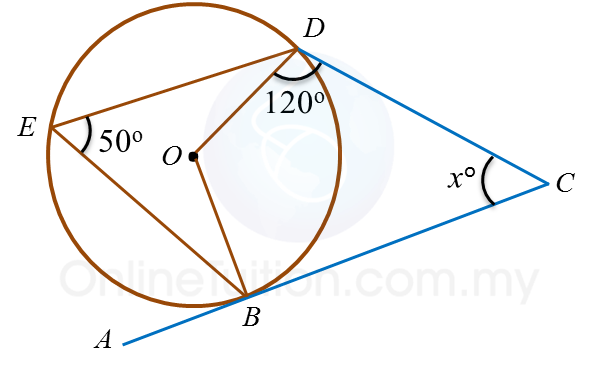# 8.4.3 Circles III, Paper 1 (Short Questions)

8.4 Circles, SPM Practice (Short Questions)
Question 9:In figure above, ABC is a tangent to the circle centre O at B. AED is a straight line.
Find the value of y.

Solution:
ABO = 90o
BOE = 2 × 40o = 80o
AEO = 360– ∠ABO  – ∠BOE – 35o
= 360– 90o – 80o – 35= 155o
yo+ ∠AEO = 180
yo+ 155o = 180o
y= 180o  – 155o
y o = 25

Question 10:In figure above, ABC is a tangent to the circle centre O, at point B.
The value of is

Solution:
OBC = 90
BOD = 2 × 50o = 100o